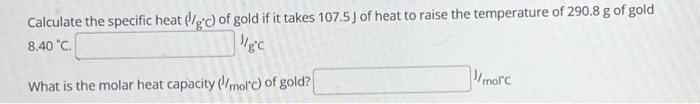Home / Expert Answers / Chemistry / calculate-the-specific-heat-gc-of-gold-if-it-takes-107-5-j-of-heat-to-raise-the-temperature-of-290-pa193

# (Solved): Calculate the specific heat (gc) of gold if it takes 107.5 J of heat to raise the temperature of 290 ...

Calculate the specific heat (gc) of gold if it takes 107.5 J of heat to raise the temperature of 290.8 g of gold 8.40 °C. J/gc What is the molar heat capacity (J/mol'c) of gold? J/mol'cCalculate the specific heat of gold if it takes of heat to raise the temperature of of gold J J/ What is the molar heat capacity of gold? molc

We have an Answer from Expert